Announcement: We're launching LabelGPT, World's fastest prompt based labeling tool. Join the waiting list to get beta access

# What's GAN (generative adversarial networks), how it works?

Have you ever considered transforming your zebra to horse or horse to zebra images? Have you ever considered foreseeing the following video frame? Imagine being able to create an image from a sentence.

Would you like to create emojis using images? Do you want to make an image from various perspectives or poses? What if you could transform a daytime scene into a nighttime one?

One of the most talked-about issues in deep learning is generative adversarial networks (GANs). Unsupervised learning is carried out using Generative Adversarial Networks, or GANs. The number of GAN applications has greatly increased. Understanding generative adversarial networks will help us better understand how they operate.

## What is GAN?

In a generative adversarial network (GAN), two neural networks compete with one another to make predictions that are as accurate as possible. GANs typically operate unsupervised and learn through cooperative zero-sum games.

The generator and the discriminator are the two neural networks that constitute a GAN. A de-convolutional neural network serves as the discriminator, and a convolutional neural network serves as the generator.

The generator's objective is to provide outputs that could be mistaken for actual data by users. The discriminator's objective is to determine whether the outputs it receives were produced intentionally.#### Train your data with Labellerr

Automate any image annotation project in a few clicks to save time and reduce cost.

## Different Types of GANs

There are many different forms of GAN implementation, and GAN research is now quite active. Following is a list of some of the significant ones that are now in use:

Vanilla GAN: The most basic GAN type is called a vanilla GAN. The Generator and Discriminator in this scenario are straightforward multi-layer perceptrons. Simple stochastic gradient descent is used in vanilla GAN's approach to try and optimize the mathematical problem.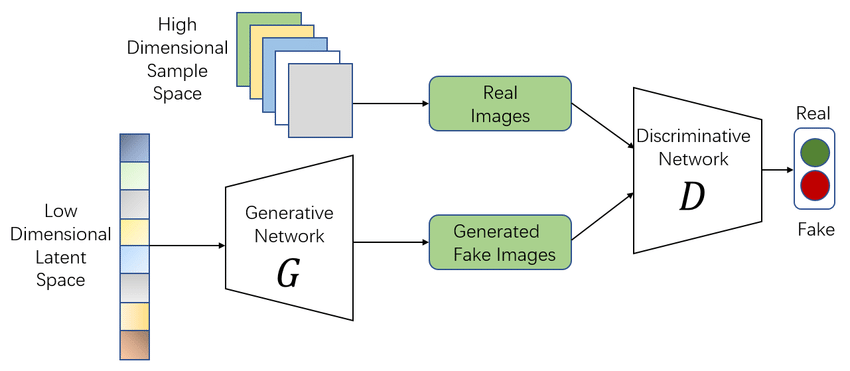Conditional GAN (CGAN): CGAN is a deep learning technique that employs a number of conditional parameters. In CGAN, the Generator is given an extra parameter, "y," to produce the necessary data. Labels are also included in the Discriminator's input so that it can help distinguish between authentic data and artificially generated data.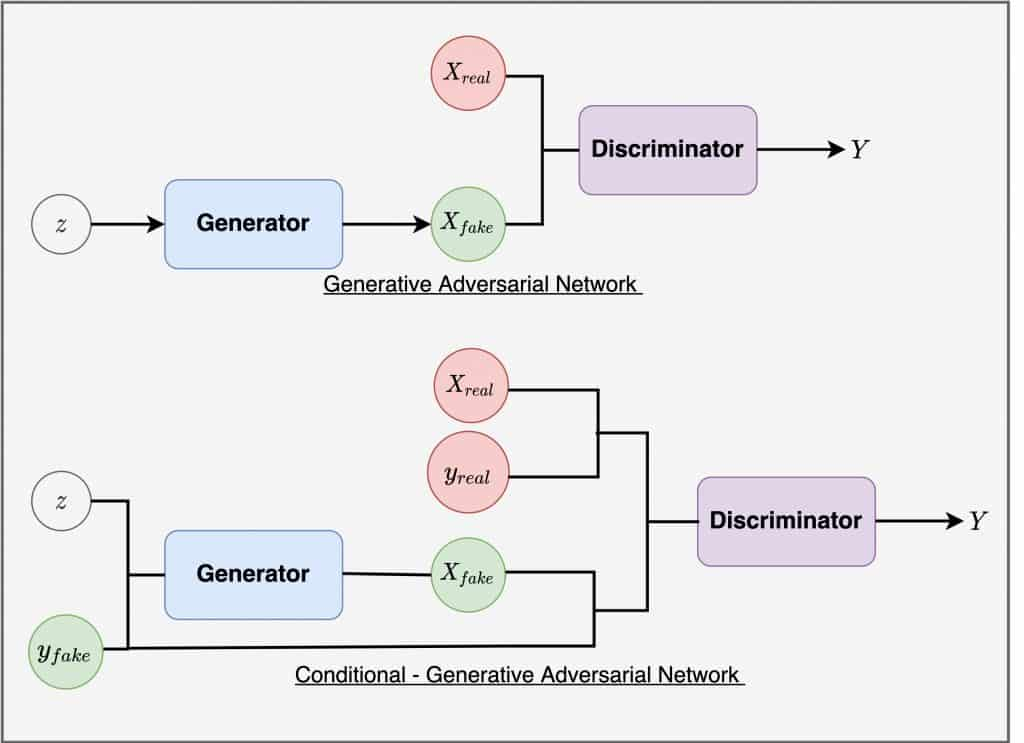Deep Convolutional GAN (DCGAN): DCGAN is among the most well-liked and effective GAN implementations. ConvNets are used instead of multi-layer perceptrons in their construction. Convolutional stride actually replaces max pooling in the ConvNets implementation. The layers are also not entirely connected.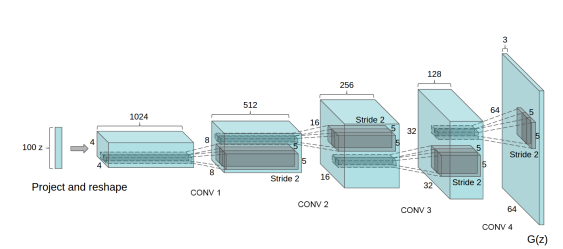Super Resolution GAN (SRGAN): As the name implies, SRGAN is a method for creating a GAN that uses both a deep neural network and an adversarial network to generate higher-resolution images. This particular sort of GAN is very helpful in enhancing details in native low-resolution photos while minimizing mistakes.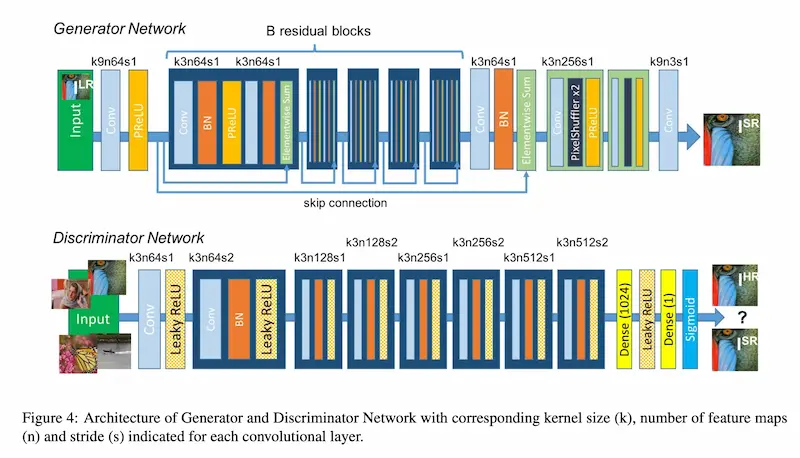Laplacian Pyramid GAN (LAPGAN): A set of band-pass pictures that are separated by an octave and a low-frequency residual make up the Laplacian pyramid, a linear invertible image representation. This method makes use of several Generator and Discriminator networks as well as various Laplacian Pyramid levels.

The major reason this method is employed is that it results in photographs of extremely high quality. The image is initially downscaled at each pyramidal layer before being upscaled at each layer once again in a backward pass. At each of these layers, the image picks up noise from the Conditional GAN until it reaches its original size.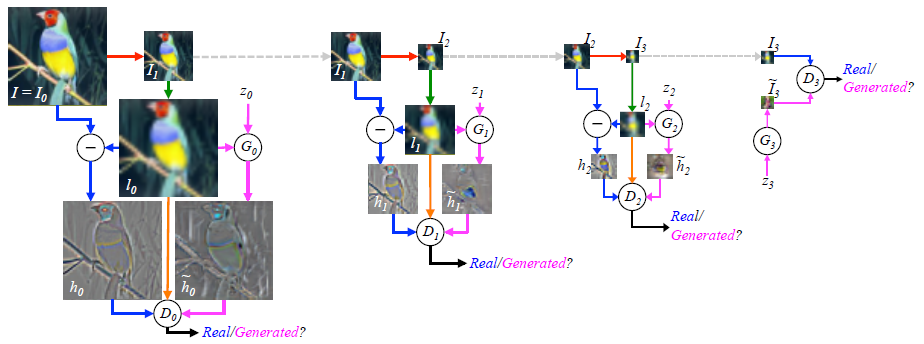## Why did GANs initially come into being?

Most common neural networks have been found to be easily tricked into classifying objects incorrectly by introducing just a tiny bit of noise into the initial data. Surprisingly, the model has greater confidence in the incorrect forecast after noise addition than it does in the accurate prediction.

Due to the fact that most machine learning models only learn from a small amount of data—a major disadvantage that makes them vulnerable to over-fitting—this enemy exists. Additionally, the input-to-output mapping is nearly linear.

Despite the fact that it may appear that the boundaries separating the different classes are linear, they are actually constructed of linearities, and even a little modification in a point in the higher dimensional space could result in incorrect data categorization.

## How do GANs Work?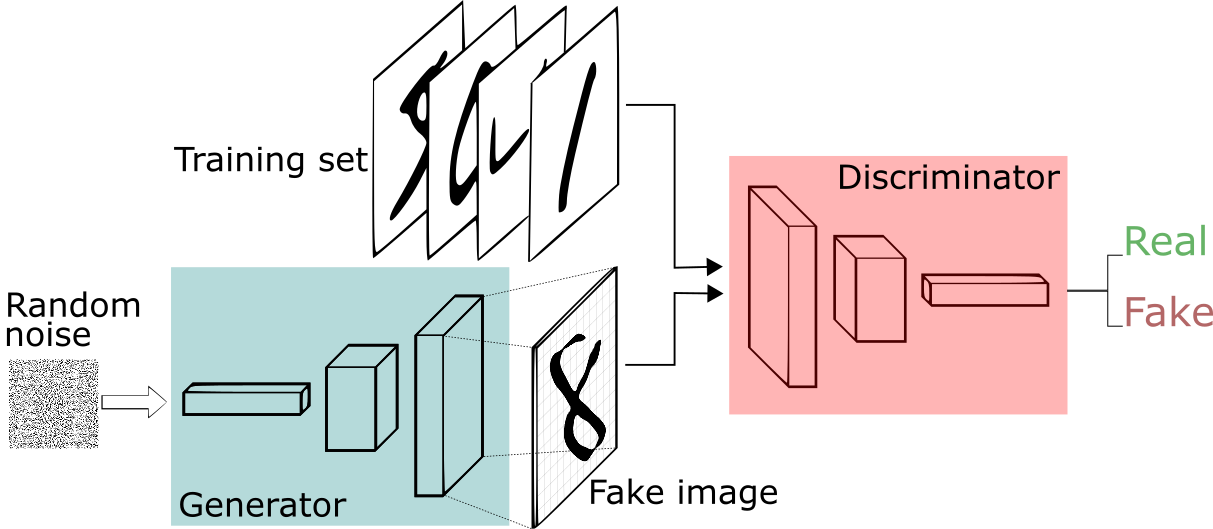The discriminator determines if each instance of data that it analyzes is actually a part of the training data set, whereas the generator, also known as the generator, creates new data instances, and the discriminator, also known as the discriminator, evaluates them for authenticity.

Say our goal is to imitate something less complex than the Mona Lisa. We'll create handwritten numbers that resemble those in the MNIST dataset, which is derived from data collected in the actual world. When the discriminator is provided with an instance from the real MNIST data set, its objective is to identify those that are genuine.

The discriminator receives fresh, synthetic images from the generator, which is making them in the background. It does this with the expectation that even if they are fraudulent, they will also be accepted as legitimate. The generator's objective is to produce plausible handwriting that can be used to cover up lies. The discriminator's objective is to reveal bogus images produced by the generator.

The following are the actions a GAN takes:

• The generator outputs an image after receiving random numbers.
• The discriminator receives this created image in addition to a stream of photos from the real, ground-truth data set.
• The discriminator inputs both authentic and fraudulent images and outputs probabilities, a value between 0 and 1, with 1 denoting a prediction of authenticity and 0 denoting fraudulent.

A GAN can be compared to the cat-and-mouse game between a cop and a counterfeiter, in which the counterfeiter learns to pass fake currency and the cop learns to spot it.

Both are dynamic; for example, the cop is learning as well (to continue the analogy, perhaps the central bank is flagging banknotes that snuck through), and each side continuously escalates their learning of one another's strategies.

The discriminator network used by MNIST is a common convolutional network that can classify the images supplied to it, acting as a binary classifier that labels images as authentic or fraudulent.

In a sense, the generator is an inverted convolutional network: instead of down-sampling an image to create a probability as in a conventional convolutional classifier, the generator resamples a vector of random noise to create an image. The second creates new data while the first discards data through down-sampling methods like max pooling.

In a zero-sum game, each net is attempting to maximize a different and opposing objective function, or loss function. Essentially, this is an actor-critic model. The generator and discriminator both modify their behavior as the discriminator does. Their losses conflict with one another.

## Costs of the Generator and Discriminator

The cost of each participant determines how the algorithm will proceed. Using the Minimax game, where the generator value is equal to the discriminator cost minus one, is the simplest way to define the cost.

What precisely is this cost that the generator would like to minimize and the discriminator to maximize?

The relationship between the output of the discriminator and the real labels (real/fake) is essentially a regular cross-entropy function.

The discriminator would seek to maximize the log-likelihood of predicting one, demonstrating that the input is real, while the first term in J(D) symbolizes feeding the discriminator the actual data. The samples produced by G are represented by the second phrase. In this situation, the discriminator would aim to maximize the log-likelihood of correctly predicting zero, which would indicate that the data is bogus.

On the other hand, the generator seeks to reduce the log chance of the discriminator being accurate. The saddle position of the discriminator loss, which is the equilibrium aim of the game, is the answer to this conundrum.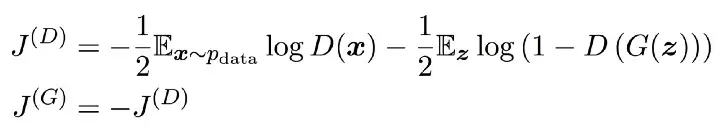The primary issue with this Minimax game is that as the discriminator gets smarter, the gradient for the generator disappears. Instead of only reversing the value of the discriminator's cost, this can be fixed by flipping the order of the inputs in the cross-entropy function.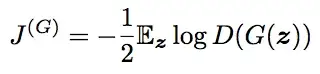The goal of the generator at this point would be to maximize the log-likelihood that the discriminator is incorrect. Now that the equilibrium cannot be captured by a single value function, it is much more heuristic to justify this particular cost.